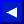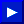If an array referenced in a program was never DiMensioned, it is automatically dimensioned to 11 elements in each dimension used in the first reference.

EXAMPLES of DIM Statement:
```   10 DIM A(100)
20 DIM Z (5,7), Y(3,4,5)
30 DIM Y7%(Q)
40 DIM PH\$(1000)
50 F(4)=9 : REM AUTOMATICALLY PERFORMS DIM F(10)
```
EXAMPLE of FOOTBALL SCORE-KEEPING Using DIM:
```   10 DIM S(1,5), T\$(1)
20 INPUT"TEAM NAMES"; T\$(0), T\$(1)
30 FOR Q=1 TO 5: FOR T=0 TO 1
40 PRINT T\$(T),"SCORE IN QUARTER" Q
50 INPUT S(T,Q): S(T,0)= S(T,0)+ S(T,Q)
60 NEXT T,Q
70 PRINT CHR\$(147) "SCOREBOARD"
80 PRINT "QUARTER"
90 FOR Q= 1 TO 5
100 PRINT TAB(Q*2+9) Q;
110 NEXT: PRINT TAB(15) "TOTAL"
120 FOR T=0 TO 1: PRINT T\$(T);
130 FOR Q= 1 TO 5
140 PRINT TAB(Q*2+9) S(T,Q);
150 NEXT: PRINT TAB(15) S(T,0)
160 NEXT
```
CALCULATING MEMORY USED BY DIM:
5 bytes for the array name
2 bytes for each dimension
2 bytes/element for integer variables
5 bytes/element for normal numeric variables
3 bytes/element for string variables
1 byte for each character in each string elementThis page has been created by Sami Rautiainen. Read the small print. Last updated November 08, 1998.